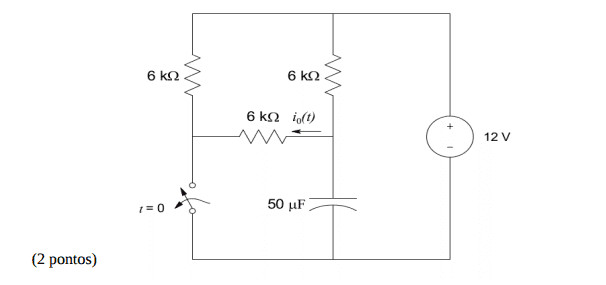# Help calculating the voltage in a capacitor when t->infinite

• lucasLima

#### lucasLima

Member advised to use the homework template for posts in the homework sections of PF.Hello Everyone!
So, I need to calculate the voltage in the capacitor, let's call it Vc(t).

For t=0- I know that there is no current in the capacitor. Did some calculations and I've found that Vc(0)=6v.
Now i need to know Vc(infinite)

But when the switch key is opened and the time goes to inifinte there is no current going through right? Because the capacitor works as an open circuit when there is no change.
So, is Vc(inifinite)=0?

But when the switch key is opened and the time goes to inifinte there is no current going through right? Because the capacitor works as an open circuit when there is no change.
So, is Vc(inifinite)=0?
It won't be infinite. There's no potential source in the circuit that's that large!

edit: Oops. My apologies, I misread your statement. No, the potential across the capacitor will not be zero.

Suppose you were to remove the capacitor from the circuit and replace it with an ideal voltmeter. What would it read?

Hint: With the switch open and the capacitor removed you should be able to simplify the resistor network. Maybe that will help you see the answer...

Last edited:
When t=∞ the current into/out of the capacitor becomes zero, meaning there will be no current to the capacitor via the resistor network. No current implies no voltage loss across the resistor feeding into the capacitor.

You could imagine removing the capacitor, and seeing what voltage exists where its terminals were; that's the voltage it will charge to by t=∞.